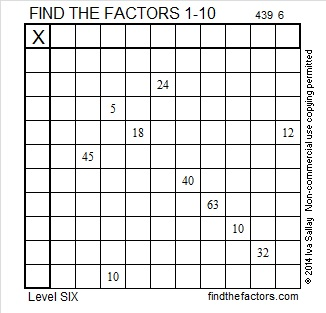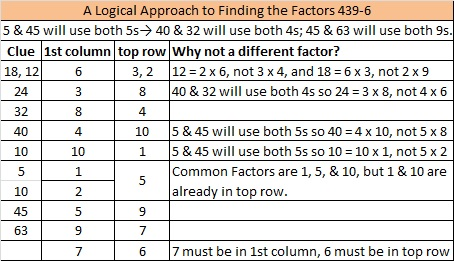# 251 and Level 6

• 251 is a prime number.
• Prime factorization: 251 is prime.
• The exponent of prime number 251 is 1. Adding 1 to that exponent we get (1 + 1) = 2. Therefore 251 has exactly 2 factors.
• Factors of 251: 1, 251
• Factor pairs: 251 = 1 x 251
• 251 has no square factors so its square root cannot be simplified. √251 ≈ 15.843How do we know that 251 is a prime number? If 251 were not a prime number, then it would be divisible by at least one prime number less than or equal to √251 ≈ 15.843. Since 251 cannot be divided evenly by 2, 3, 5, 7, 11, or 13, we know that 251 is a prime number.Print the puzzles or type the factors on this excel file: 10 Factors 2014-09-29This site uses Akismet to reduce spam. Learn how your comment data is processed.